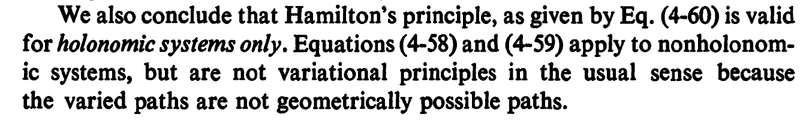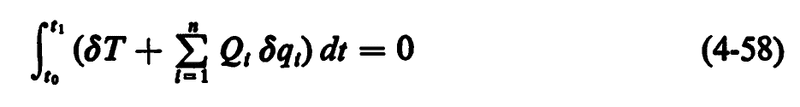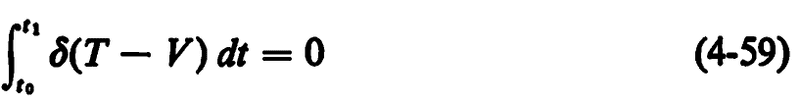# When is Hamilton's principle valid?

• A
Kashmir
When is Hamilton's principle##\delta \int L d t=0## valid ?
Is it only valid for monogenic and holonomic systems? What about monogenic and non holonomic systems?

(I'm asking this because I got confused because I've found that Goldstein has got something wrong related to this in his 3rd edition)

•Delta2

Gold Member
2022 Award
I don't know, what a monogenic system is, but it's also valid for non-holonomic systems. The distortion of Goldstein's book is only for the 3rd and higher editions. The 2nd is correct, leading to the same equations of motion for non-holonomic systems using correctly Hamilton's action principle as you get with d'Alembert's principle, which are equivalent for forces which have a (generalized) potential.

•Delta2
Is it only valid for monogenic and holonomic systems?
yes it is, for monogenic and holonomic systems only
See for example
Bloch, A.M., J. Ballieul, P. Crouch and J. E. Marsden , Nonholonomic Mechanics and Control

or Greenwood_D.T. Classical_dynamics

Last edited:
Gold Member
2022 Award
Is d'Alembert's principle also invalid for non-holonomic systems? What does "monogenic" mean. I've never heard this expression before.

andresB
Is d'Alembert's principle also invalid for non-holonomic systems? What does "monogenic" mean. I've never heard this expression before.

Monogeneic means that all the (non-constraint) forces comes from a generalized potential.

•Kashmir and vanhees71
Is d'Alembert's principle also invalid for non-holonomic systems?
d'Alembert's principle is valid for non-holonomic systems. We have been arguing this topic for a long time but you have never read texts I referred to.

andresB
If I remember correctly, last week I read an article that says that Hamilton's principle work just fine for non-holonomic if and only if the constraint equations are linear in the velocities. But I can't find the pdf at the moment.

Gold Member
2022 Award
d'Alembert's principle is valid for non-holonomic systems. We have been arguing this topic for a long time but you have never read texts I referred to.
I don't have these texts, but I don't understand this claim, because with the correct use of the constraints for the variations you get the same equations of motion, the Euler-Lagrange equations of the first kind with Lagrange multipliers as with the analogous calculation using d'Alembert's principle. Thus, either both d'Alembert's and Hamilton's principle are applicable to non-holonomic constraints or both are not applicable.

QM is not my cup of tea,
please formulate this variational principle here in the general analysis terms that are independent on QM

andresB
QM is not my cup of tea,
please formulate this variational principle here in the general analysis terms that are independent on QM
The thing about that work is that it is 100% classical physics, and no quantum mechanics is involved.

It is hard to give a translation to the standard analysis or to calculus of variations in manifolds, that's mostly still an open problem.

Kashmir
yes it is, for monogenic and holonomic systems only
See for example
Bloch, A.M., J. Ballieul, P. Crouch and J. E. Marsden , Nonholonomic Mechanics and Control

or Greenwood_D.T. Classical_dynamics
And we can extend it to one special case of non holonomic case as shown below?
If I remember correctly, last week I read an article that says that Hamilton's principle work just fine for non-holonomic if and only if the constraint equations are linear in the velocities. But I can't find the pdf at the moment.

That's also in Goldstein. He too uses Hamilton's principle for a special non holonomic case where we have
##
\sum_{k} a_{l k} d q_{k}+a_{t t} d t=0
##.

And we can extend it to one special case of non holonomic case as shown below?
Greenwood mentioned above:•Kashmir
Gold Member
2022 Award
This is simply wrong! I don't know when the textbook writers started to make these wrong statements leading to the wrong vakonomic dynamics. In all traditional textbooks the d'Alembert principle and the action principle are equivalent for nonholonomic constraints. The only restriction for the action principle is that the forces should be derivable from a generalized potential.

The correct way to implement the nonholonomic constraints, which are by assumption of the form
$$A_{ak}(q,t) \dot{q}^k+B_a(q,t)=0.$$
Here the ##k \in \{1,\ldots,n \}## labels the generalized coordinates and ##a \in \{1,\ldots,r \}## the different constraints. The Einstein summation convention is used.

In form of differentials the constraints read
$$A_{ak}(q,t) \mathrm{d} q^k + B_a(q,t) \mathrm{d} t=0.$$
For the action principle we assume that
$$L=L(q,\dot{q},t),$$
and the action
$$A[q]=\int_{t_1}^{t_2} \mathrm{d} t L(q,\dot{q},t)$$
should be stationary under variations ##\delta q##, ##\delta t=0## (the latter is the important point here!). The variations of the ##\delta q## are however not independent but constrained by the in general nonholonomic constraints. Since ##\delta t=0## these constraints read
$$A_{ak}(q,t) \delta q^k + B_a(q,t) \delta t=A_{ak}(q,t) \delta q^k=0.$$
We can vary the ##\delta q^k## arbitrarily and take the constraints on the variation into account by introducing ##r## Lagrange multipliers ##\lambda_a##, i.e., we make
$$\delta A+\int_{t_1}^{t_2} \mathrm{d} t \lambda_a A_{ak}(q,t) \delta q^k=0.$$
The variation of ##A## reads, using that by definition the initial and final points have to be kept fixed in Hamilton's principle, ##\delta q^k(t_1)=\delta q^k(t_2)=0##,
$$\delta A =\int_{t_1}^{t^2} \mathrm{d} t \left (\delta q^k \partial_{q^k} L + \delta \dot{q}^k \partial_{\dot{q}^k} L \right ) = \int_{t_1}^{t^2} \mathrm{d} t \delta q^k \left [\partial_{q^k} L - \mathrm{d}_t (\partial_{\dot{q}^k} L) \right ].$$
So the stationarity of the action functional together with the Lagrange-multiplier expression for the constraints on the variations, leads to
$$\int_{t_1}^{t_2} \mathrm{d} t \delta q^k \left [\partial_{q^k} L - \mathrm{d}_t (\partial_{\dot{q}^k} L) + \lambda_a A_{ak} \right ] \stackrel{!}{=}0.$$
This leads to the equation of motion
$$\partial_{q^k} L - \mathrm{d}_t (\partial_{\dot{q}^k}) L+\lambda_a A_{ak}=0.$$
These are the same equations of motion you also get from d'Alembert's principle. These are equations for the ##n## generalized coordinates ##q^k##'s and ##r## Lagrange multipliers ##\lambda_a##. The additional equations needed to solve for these ##(n+r)## unknowns are of course the constraint equations in the original form,
$$A_{ak} \dot{q}^k+B_a=0.$$
You can find this straight-forward derivation in many classical textbooks like Landau&Lifshitz vol. 1, Goldstein (2nd condition) and, once more discussing many different principles from d'Alembert to Maupertuis, Lagrange, Hamilton, Routh, etc., including a clear treatment of the nonholonomic constraints.

So what is a stationary point of the functional and why does not it satisfy the equations of nonholonomic mechanics?

Here is a draft of a section from a short textbook which is being written by my colleague and me

#### Attachments

• lagrange.pdf
161.8 KB · Views: 56
•vanhees71
andresB
I have never noticed the order of variation and the integral in Hamiltons principle. And it is just mind-boggling. Besides Greenwood, a reference that I just read on the topic and that might be of use is L.A. Pars "A treatise on analytical mechanics" chapter XXVI.

•wrobel